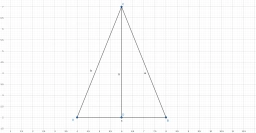# Calculation 81402

In an isosceles triangle, the side a=b= 21 cm, and the triangle's height is 19 cm. Find out the base and perimeter of the triangle (sketch, calculation, answer).

c =  17.8885 cm
o =  59.8885 cm

### Step-by-step explanation:Did you find an error or inaccuracy? Feel free to write us. Thank you!

Tips for related online calculators
The Pythagorean theorem is the base for the right triangle calculator.
Calculation of an isosceles triangle.